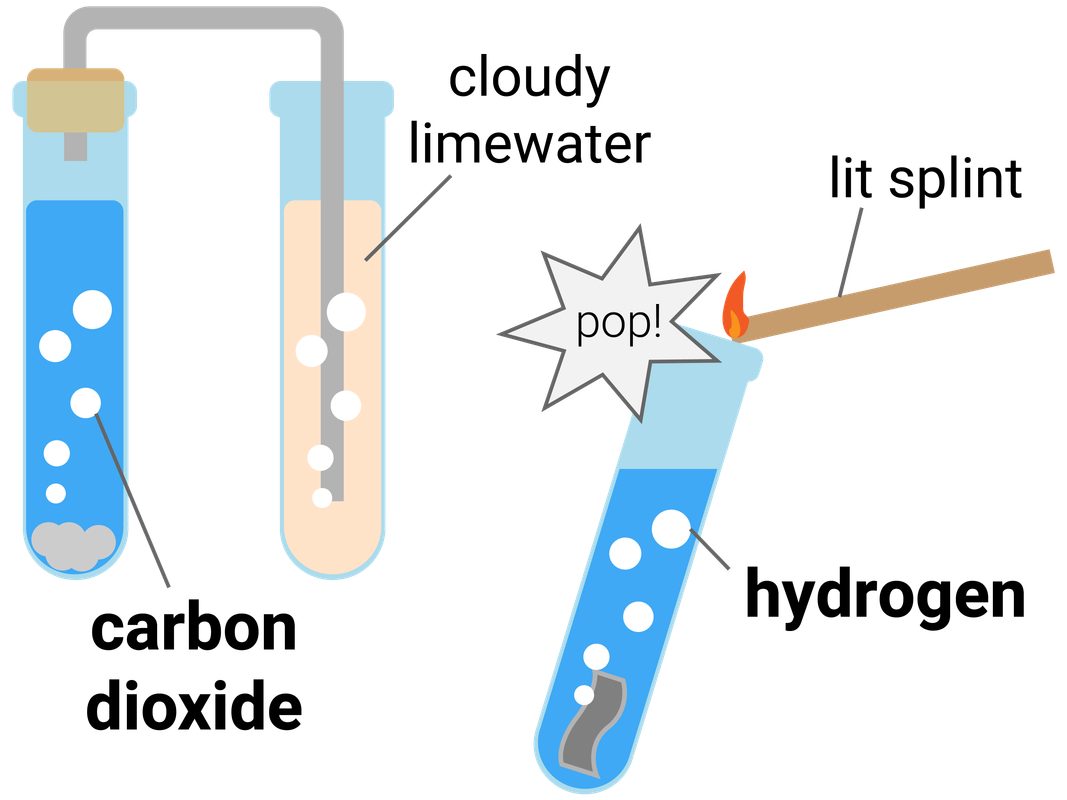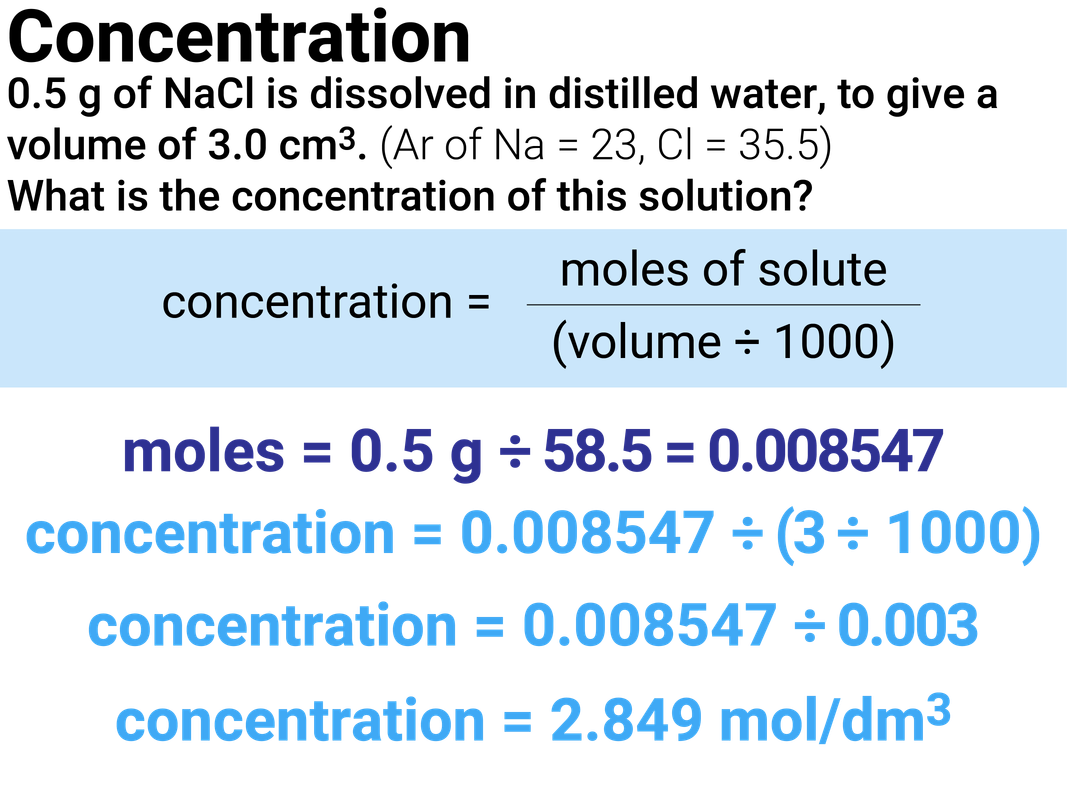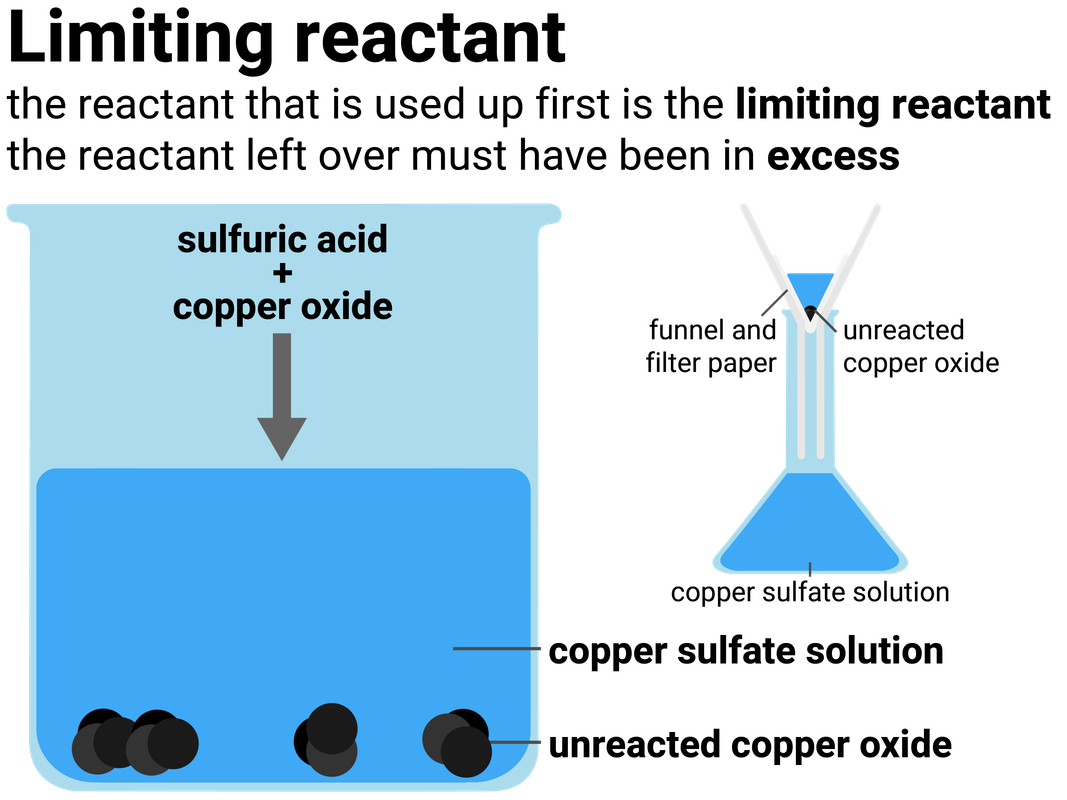# Introducing Chemical Reactions

### Conservation of Mass

The law of conservation of mass states that no atoms are created or destroyed during a chemical reaction, they are only rearranged. This means that the mass of the products must be the same as the mass of the reactants.

### Closed systems

No substances can enter or exit a closed system. A simple closed system in the lab could just be a sealed flask. Sometimes reactions that happen in open beakers are still closed systems (because nothing enters or exits the system). These include:

• acid-alkali neutralisation reactions, which produce salt solutions
• precipitation reactions, which produce an insoluble precipitate (solid)

### Non-enclosed systems

Substances can enter or exit a non-enclosed system. These systems are often open flasks, or crucibles, that let gases enter or exit.

If a gas escapes, it can look like the total mass has decreased. If a gas is added, the total mass will look as if it has increased. However, the total mass stays the same if the mass of the gas is included in calculations.### Half Equations

Higher Tier

A half equation shows what happens in reactions involving ions in terms of the electron transfer. It shows what happens when ions gain electrons (reduction) or lose electrons (oxidation). In half equations:

• electrons are shown as e-
• the numbers of atoms of each element must be the same on both sides (like in a balanced equation)
• the total charge on each side needs to be the same

### Negative ions

The most common half equations you will need to write a half equation for include: halides, oxygen, hydroxide, or a metal.

2Cl-(aq) - 2e- → Cl2(g)
2O2-(aq) - 4e- → O2(g)
4OH-(aq) - 4e- → 2H2O(l) + O2(g)
Cu(s) - 2e- → Cu2+(aq)

### Positive ions

Group one metals, and hydrogen, only have a +1 charge, and so only need to gain one electron, but notice how because hydrogen gas is made of two hydrogen atoms, we must start with 2 hydrogen ions:

Na+(aq) + e- → Na(s)
K+(aq) + e- → K(s)
2H+(aq) + 2e- → H2(g)
Cu2+(aq) + 2e- → Cu(s)

### Ionic equations

Ionic equations show the ions that are involved in a chemical reaction (removing any ions that do not change, refered to as spectator ions). They can either be written by simplifying a balanced symbol equation, or by adding two half equations together.

turning a balanced symbol equation:
Mg(s) + CuSO4(aq) → MgSO4(aq) + Cu(s)
or, when rewritten in terms of ions:
Mg(s) + Cu2+(aq) SO42-(aq) → Mg2+(aq) SO42-(aq) + Cu(s)

or adding two half equations together:
Mg(s) - 2e- → Mg2+(aq)
Cu2+(aq) + 2e- → Cu(s)

becomes this ionic equation:
Cu2+(aq) + Mg(s) → Cu(s) + Mg2+(aq)

### Hydrogen

The test for hydrogen uses a burning splint held at the open end of a test tube of the gas. Hydrogen burns rapidly with a pop sound.

This test requires you to allow a second, or two, for oxygen gas from the air to mix with the hydrogen first, as the test is actually showing the chemical reaction between hydrogen and oxygen to make water.

### Carbon Dioxide

The test for carbon dioxide uses an aqueous solution of calcium hydroxide (limewater). When carbon dioxide is shaken with or bubbled through limewater the limewater turns milky (cloudy).

This test is actually a reaction between the carbon dioxide and limewater, to make limestone (calcium carbonate).

### Oxygen

The test for oxygen uses a glowing splint inserted into a test tube of the gas. The splint relights in oxygen.

### Chlorine

The test for chlorine uses litmus paper. When damp litmus paper is put into chlorine gas the litmus paper is bleached and turns white.### The Mole

Higher Tier

Chemical amounts are measured in moles (symbol: mol). The mass of one mole of substance in grams is equal to the substance’s RAM/RFM. One mole of a substance contains the same number of particles, atoms, molecules or ions as one mole of any other substance.

The number of atoms, molecules or ions in a mole of a given substance is the Avogadro constant. The value of the Avogadro constant is 6.02×10²³ per mole. This means that for every one mole of substance (no matter the substance), it will always contain 6.02×10²³ particles.

name of
element
relative
atomic mass
1 mole
(mass)
1 mole
(particles)
carbon 12 12 g 6.02×10²³
sodium 23 23 g 6.02×10²³

moles = mass (g) ÷ RFM

We can use this equation to calculate the number of moles of a chemical in a given amount of mass.Worked Example 1

Calculate the number of moles in 20 g of MgO
(RAM: Mg=24, and O=16)

MgO has an RFM of 40
we can calculate that there are 0.5 moles of MgO in 20 g by dividing the mass by the RFM (20 ÷ 40)

Worked Example 2

Calculate the number of moles of oxygen needed to make 6 moles of magnesium oxide.
(RAM: Mg=24, and O=16)

2Mg + O2 → 2MgO

For every 1 mole of O2 we make 2 moles of MgO
we made 6 moles of MgO so we need 3 moles of O2 (6 ÷ 2)

Worked Example 3

Calculate the number of moles of magnesium oxide created, when 12 g of magnesium is burned in air.
(RAM: Mg=24, and O=16)

2Mg + O2 → 2MgO

For every 2 moles of Mg we make 2 moles of MgO
we have 0.5 moles of Mg (12 ÷ 24) so we make 0.5 moles of MgO
MgO has an RFM of 40...
so we make 20 g of MgO (0.5 × 40)

### Concentration

solution is formed when a solute dissolves in a solvent. The concentration of a solution is a measure of the mass, or amount of solute, dissolved in a given volume of solvent. The more concentrated the solution, the more particles it contains in a given volume.

### Calculating concentration

The concentration of a solution can be calculated using:

• the mass of dissolved solute (in g)
• the volume of solvent (in dm3)

concentration = mass of solute  ÷ volume of solvent

The units for concentration are g/dm3, but they may also be written as gdm-3 - don't worry as these mean the same thing.

### Volume unit conversions

Sometimes volumes can be quoted in ml, rather than cm3. These units describe the same volume. For example, 250 ml = 250 cm3.
If your volume is given in cm3, then you will need to convert it into dm3 before it can be used in this calculation.

• divide by 1000 to convert from cm3 to dm3
• multiply by 1000 to convert from dm3 to cm3### Higher Tier

The concentration of a solution can be calculated using:

• the moles of dissolved solute (in mol)
• the volume of solvent (in dm3)

concentration = moles of solute  ÷ volume of solvent

The units for concentration are mol/dm3, but they may also be written as moldm-3 - don't worry as these mean the same thing.

### Converting from grams to moles

You need to be able to calculate concentration in gdm-3 and be able to convert these units into mol/dm3.

To do this, think back to our moles equations, where:

moles = mass ÷ Mr

For example, a solution of sodium hydroxide has the concentration of 5 g/dm3.
To convert it into mol/dm3, we divide 5 ("the mass") by 40 (the Mr of NaOH).
This gives a concentration of 0.125 mol/dm3.### Limiting Reactants

Higher Tier

Reactions stop when one of the reactants is used up. This is because the other reactant has nothing more to react with, and so some of it will be left over.

The substance that runs out is called the limiting reactant. The one left over we say has been added in excess.

The stoichiometry of a reaction is the ratio of the amounts of each substance in the balanced equation. It can be worked out using masses found by experiment.

action Mg O2
calculate amounts
0.6 ÷ 24 = 0.025 mol 0.2 ÷ 16 = 0.0125 mol
Divide by the smallest number 0.25 ÷ 0.0125 = 2 0.125 ÷ 0.0125 = 1

This means that 2 moles of Mg reacts with 1 mol of O2, so the left-hand side of the equation is: 2Mg + O2

Then balancing the equation gives us: 2Mg + O2 → 2MgO